# Calibrating a Power Supply with a Digital Potentiometer

### Abstract

A resistive feedback network is often used to set the output voltage of a power supply. A mechanical potentiometer (pot) conveniently solves the problem of adjusting a power supply. For easier automatic calibration, a mechanical pot can be replaced with a digital pot. This application note presents a calibration solution that uses a digital pot, because digipots are smaller, do not move with age or vibration, and can be recalibrated remotely. This proposed solution reduces the susceptibility of the system to the tolerance of the digital pot's end-to-end resistance, making the solution optimal for designers. This application note also explains some of the equations required to calculate the resistor chain values and to use a digital pot in this way. A spreadsheet with standard reisistor values is available for easy calculations.

A similar version of this article appeared on Power Management DesignLine, February 2012.

### Introduction

A resistive feedback network is often used to set the output voltage of a power supply, since fixed resistors are simple to use and low cost. However, because a fixed network is not adjustable, the output voltage cannot be accurately set. Therefore, many power supplies use a mechanical potentiometer (pot) in the feedback network to set the output voltage. For easier automatic calibration, a mechanical potentiometer can be replaced with a digital potentiometer. Digital potentiometers are smaller, do not move significantly with age or vibration, and can even be recalibrated remotely. This application note explains some of the calculations required to use a digital potentiometer in this way and also provides a spreadsheet for easy calculations.

### Conventional Power-Supply Feedback

A power supply often uses resistive feedback to set the output level. However, because there are many device tolerances that can affect the output voltage, a fixed resistor-divider is often insufficient. In these situations, a variable-resistor ratio is used so the system can be calibrated in final testing.
Although a mechanical potentiometer would have traditionally been used, those components have been replaced by digital potentiometers in many applications. Digital pots do not suffer from significant aging effects and are far simpler to adjust automatically in final tests.
Fixed-resistor and mechanical-potentiometer schemes are shown in Figures 1 and 2.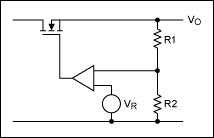Figure 1. A conventional fixed-resistive feedback.Figure 2. A conventional variable-resistive feedback.
Take the typical example of a 12V supply with a 2.5V reference voltage. The reference voltage and all other devices in the system will have a tolerance. Conveniently, all tolerances can be represented as additional tolerance on the reference. For this example, we will use a ±5% typical total tolerance for the reference.
Calculating the values for a fixed resistive feedback is based on an easy equation:(Eq. 1)
Since in this example, VR will have a tolerance of ±5%, this leads to a tolerance in the output voltage of 5%. If this is not acceptable in the application, the resistive-divider needs to be made variable. Replacing R1 and R2 with a mechanical potentiometer is not normally done, since it would result in a wide range of output voltages and would be very sensitive to adjustments. Over time and temperature, any drift in the pot's position would create an unacceptable amount of output voltage drift. Therefore, Figure 2 illustrates a scheme that reduces the output range, which is now easier to adjust and is more stable.
Hypothetically, we should be able to replace R2 with a digital pot, and we should have an electrically adjustable system. However, it is not as simple as that. First, some explanation of digital pots is required.

### Basic Structure of Digital Pots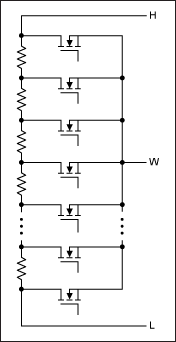Figure 3. Typical digital potentiometer structure.
A digital pot is normally a string of resistors with a switch at each node, as shown in Figure 3. For simplicity, the switches are shown as single MOSFETs. Typically, these switches would be two BiCMOS transistors (one "P" and one "N") to produce low on-resistances.
This structure is commonly used for pots up to 256 taps. When the pot is above 256 taps, it can be more efficient to use a more complex segmented structure. However, that is beyond the scope of this application note.
Since all resistors are manufactured on the same die, they will be very closely matched. Unfortunately, the end-to-end resistance may not be so well defined. A typical digital pot is the MAX5402, a single-channel, 256-tap device with end-to-end resistance of 10kΩ (typ). The end-to-end resistance tolerance, however, is ±20%. Ratiometrically, it is far better defined with integral nonlinearity (INL) of 0.5 LSB (max). It is, therefore, well suited to be used as a potential divider.

### Using the Digital Pot to Calibrate a Power Supply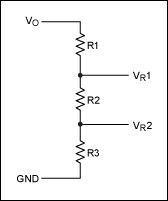Figure 4. The initial resistor string.Figure 5. The final resistor string.
The method to calculate the resistor values R1, R2, and R3, is shown in Figure 4. We will use the following example:
VO = 12V
VR = 2.5V ±5%
RT = R1 + R2 + R3
The initial equations are simple and defined below:(Eq. 2)(Eq. 3)
 R1 = RT - R3 - R2 (Eq. 4)
The first step is to define a total resistance for RT, using (R1 + R2 + R3). Since this is arbitrary, we'll start with RT = 20kΩ. (We can always change it later to give more realistic values for R1, R2 and R3 if required.) From Equation 2, we find R3 = 3.598kΩ. From Equation 3, R2 = 417Ω, and from Equation 4, R1 = 15.625kΩ.
Clearly the ideal resistor values calculated will not generally be available, so standard resistor values need to be used. The closest 1% values are substituted for R1 and R3: R1 = 15.8kΩ, R3 = 3.92kΩ. (For convenience, we have included standard resistor value charts in the spreadsheet.)
Now we can calculate backward to find an ideal value for R2, as shown in Equation 5. R2 will eventually become variable. Thus, its value is calculated so that VO will be correct when R2 is cantered and VR is at its nominal value.(Eq. 5)
Therefore, R2 = 646Ω.
We need to account for the fact that digital pots have a very poor end-to-end tolerance. Using a large-value digital pot in parallel with a small-value fixed resistor is a simple method of reducing the effect of the pot's poor end-to end tolerance. This is illustrated in Figure 5. Thus, the parallel combination of R2A and RP makes R2.
Using Equation 6, the final step is to calculate the value of the parallel fixed resistor, given a potentiometer nominal end-to-end resistor.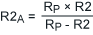(Eq. 6)
So, using a 10kΩ pot for RP, the ideal value for R2A is 690Ω. The closest 1% value is 698Ω. If we calculate the parallel combination of this and the digital pot at its tolerance extremes, we get RMIN = 642Ω and RMAX = 660Ω. This is a tolerance of only 1%, due to the 20% end-to-end tolerance of the pot. We use a 698Ω resistor for R2A, as this is the closest standard 1% value.
The final calculation confirms that with real values, the digital pot can cover the required range of 5% for the reference tolerance. We can use the star-delta transformation, as shown in Figure 6. We obviously do not need to calculate R6.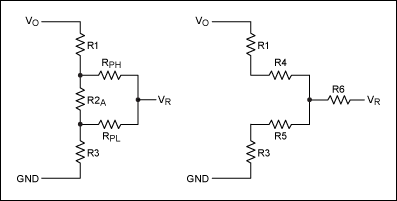Figure 6. Using the star-delta transformation.(Eq. 7)
Where N is the tap position and NMAX is the maximum tap position.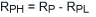(Eq. 8)(Eq. 9)(Eq. 10)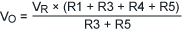(Eq. 11)
Using these equations, we confirm that the pot's range will cover the tolerance range for the reference.
To summarize the requirements and the component values selected:
VO = 12V (output voltage)
VR = 2.5V ±5% (reference voltage)
R1 = 15.8kΩ (upper resistor)
R2A = 698Ω (parallel resistor)
RP = 10kΩ (digital potentiometer)
R3 = 3.92kΩ (lower resistor)
The following tap points set the required output voltage at the extremes of reference tolerance.
VR = 2.375V, tap = 44, VO = 11.99842V
VR = 2.625V, tap = 210, VO = 11.99773V
It may be possible to optimize this further to reduce the output voltage steps. However, the potentiometer does have some overhead at either end to take account of any further tolerances.

### Summary

This application note discussed the problem of adjusting a power supply and how this problem can be solved with a mechanical pot. Additionally, the article explains a calibration solution that uses a digital pot, since digital pots are smaller, do not move with age or vibration, and can be recalibrated remotely. This proposed solution also reduces the tolerance of the digital potentiometer's end-to-end resistance, making the solution optimal for designers. The equations required to calculate the resistor chain values were developed and a spreadsheet is available that contains standard resistor value charts.